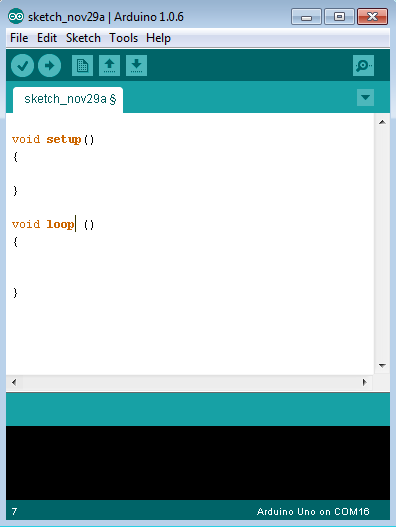# Arduino - Program Structure

In this chapter, we will study in depth, the Arduino program structure and we will learn more new terminologies used in the Arduino world. The Arduino software is open-source. The source code for the Java environment is released under the GPL and the C/C++ microcontroller libraries are under the LGPL.

Sketch − The first new terminology is the Arduino program called “sketch”.

## Structure

Arduino programs can be divided in three main parts: Structure, Values (variables and constants), and Functions. In this tutorial, we will learn about the Arduino software program, step by step, and how we can write the program without any syntax or compilation error.

Let us start with the Structure. Software structure consist of two main functions −

• Setup( ) function
• Loop( ) function```Void setup ( ) {

}
```
• PURPOSE − The setup() function is called when a sketch starts. Use it to initialize the variables, pin modes, start using libraries, etc. The setup function will only run once, after each power up or reset of the Arduino board.

• INPUT − -

• OUTPUT − -

• RETURN − -

```Void Loop ( ) {

}
```
• PURPOSE − After creating a setup() function, which initializes and sets the initial values, the loop() function does precisely what its name suggests, and loops consecutively, allowing your program to change and respond. Use it to actively control the Arduino board.

• INPUT − -

• OUTPUT − -

• RETURN − -